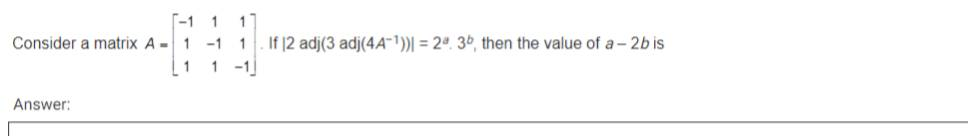# Consider a matrix A=[ matrix -1&1&1\\ 1&-1&1\\ 1&1&-1 matrix ].if|2 ad 2 adi(3 ad mathcal A ^ -1 ))|=2^ a .3^ b , then the value of a - 2b is

29 views

edited

Consider a matrix  A = $\begin{bmatrix}-1&1&1\\1&-1&1\\1&1&-1\end{bmatrix}$. If |2 adj(3 adj(4A-1))| = 2a.3b, then the value of a - 2b isby (45.9k points)
edited by

A = $\begin{bmatrix}-1&1&1\\1&-1&1\\1&1&-1\end{bmatrix}$

|A| = -1 + 1 + 1 + 1 + 1 + 1 = 4

Now,

($\because$ |KA| = Kn|A|)

= 23|3adj(4A-1)|2 ($\because$ |adj A| = |A|n-1)

= 23(33|adj (4A-1)|)2 ($\because$ |KA| = Kn|A|)

= 23.36(43. (A-1))4 ($\because$ |KA| = Kn|A|)

23.36(43. $\frac14$) ($\because$ |A-1| = $\frac1{|A|}=\frac14$)

= 23.36(42)4

= 23.36.48

= 23.36.216

= 219.36

$\therefore$ a = 19, b = 6

$\therefore$ a - 2b = 19 - 12 = 7